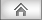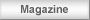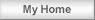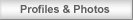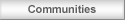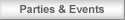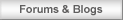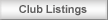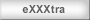Banners & Links  |  Earnings  |  Payment Details  |  Affiliates FAQ  |  NEW! Co-Branded/White Label Options! Affiliate Log-On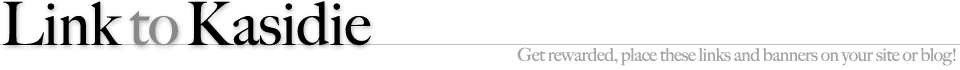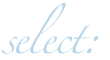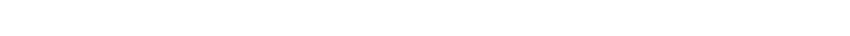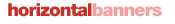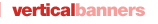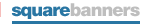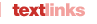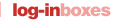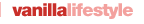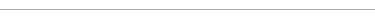Square (250 x 250)Copy and paste the following HTML code. Square (250 x 250)Copy and paste the following HTML code. Square (250 x 250)Copy and paste the following HTML code. Square (250 x 250)Copy and paste the following HTML code.
 Small Square (200 x 200)Copy and paste the following HTML code. Small Square (200 x 200)Copy and paste the following HTML code. Small Square (200 x 200)Copy and paste the following HTML code. Small Square(125 x 125)Copy and pastethe following...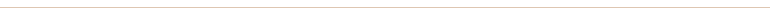Medium Rectangle (300 x 250)Copy and paste the following HTML code. Medium Rectangle (300 x 250)Copy and paste the following HTML code. Medium Rectangle (300 x 250)Copy and paste the following HTML code.Large Rectangle (336 x 280)Copy and paste the following HTML code. Large Rectangle (336 x 280)Copy and paste the following HTML code.